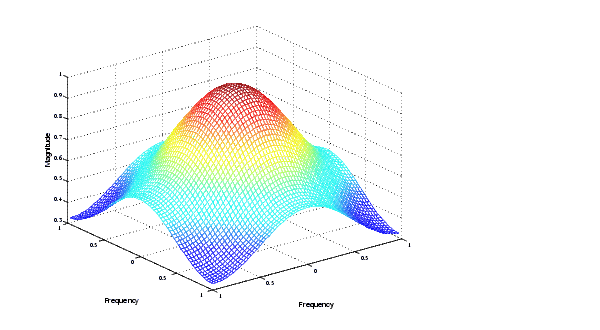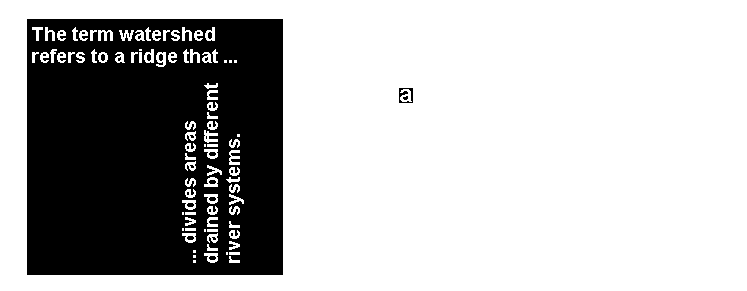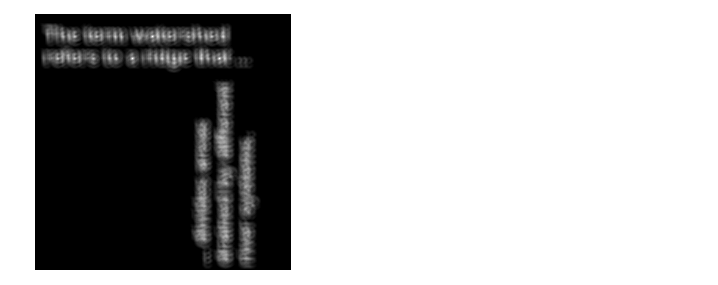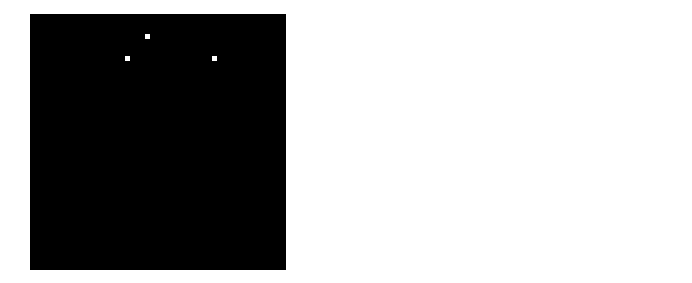Image Processing Toolbox User's GuideApplications of the Fourier Transform

This section presents a few of the many image processing-related applications of the Fourier transform.

Frequency Response of Linear Filters

The Fourier transform of the impulse response of a linear filter gives the frequency response of the filter. The function `freqz2` computes and displays a filter's frequency response. The frequency response of the Gaussian convolution kernel shows that this filter passes low frequencies and attenuates high frequencies.

• ```h = fspecial('gaussian');
freqz2(h)```

Frequency Response of a Gaussian Filter

See Linear Filtering and Filter Design for more information about linear filtering, filter design, and frequency responses.

Fast Convolution

A key property of the Fourier transform is that the multiplication of two Fourier transforms corresponds to the convolution of the associated spatial functions. This property, together with the fast Fourier transform, forms the basis for a fast convolution algorithm.

 Note    The FFT-based convolution method is most often used for large inputs. For small inputs it is generally faster to use `imfilter`.

To illustrate, this example performs the convolution of `A` and `B`, where `A` is an M-by-N matrix and `B` is a P-by-Q matrix:

1. Create two matrices.
• ```A = magic(3);
B = ones(3);
```
2. Zero-pad `A` and `B` so that they are at least (M+P-1)-by-(N+Q-1). (Often `A` and `B` are zero-padded to a size that is a power of 2 because `fft2` is fastest for these sizes.) The example pads the matrices to be 8-by-8.
• ```A(8,8) = 0;
B(8,8) = 0;
```
3. Compute the two-dimensional DFT of `A` and `B` using `fft2`.
4. Multiply the two DFTs together.
5. Compute the inverse two-dimensional DFT of the result using `ifft2`.
1. The following code performs steps 3, 4, and 5 in the procedure.

• ```C = ifft2(fft2(A).*fft2(B));
```
1. Extract the nonzero portion of the result and remove the imaginary part caused by roundoff error.
• ```C = C(1:5,1:5);
C = real(C)

C =

8.0000    9.0000   15.0000    7.0000    6.0000
11.0000   17.0000   30.0000   19.0000   13.0000
15.0000   30.0000   45.0000   30.0000   15.0000
7.0000   21.0000   30.0000   23.0000    9.0000
4.0000   13.0000   15.0000   11.0000    2.0000
```

Locating Image Features

The Fourier transform can also be used to perform correlation, which is closely related to convolution. Correlation can be used to locate features within an image; in this context correlation is often called template matching.

This example illustrates how to use correlation to locate occurrences of the letter "a" in an image containing text:

1. Read in the sample image.
• ```bw = imread('text.png');
```
2. Create a template for matching by extracting the letter "a" from the image.
• ```a = bw(32:45,88:98);
```
1. You can also create the template image by using the interactive version of `imcrop`, using the `pixval` function to determine the coordinates of features in an image.

The following figure shows both the original image and the template.

• ```imshow(bw);
figure, imshow(a);```

Image (left) and the Template to Correlate (right)

1. Compute the correlation of the template image `a` with the original image `bw` by rotating the template image by 180o and then using the FFT-based convolution technique described in Fast Convolution. (Convolution is equivalent to correlation if you rotate the convolution kernel by 180o.) To match the template to the image, use the `fft2` and `ifft2` functions.
• ```C = real(ifft2(fft2(bw) .* fft2(rot90(a,2),256,256)));
```
1. The following image shows the result of the correlation. Bright peaks in the image correspond to occurrences of the letter.

• ```figure, imshow(C,[]) % Scale image to appropriate display range.```

Correlated Image

1. To view the locations of the template in the image, find the maximum pixel value and then define a threshold value that is less than this maximum. The locations of these peaks are indicated by the white spots in the thresholded correlation image. (To make the locations easier to see in this figure, the thresholded image has been dilated to enlarge the size of the points.)
• ```max(C(:))
ans =
68.0000
thresh = 60; % Use a threshold that's a little less than max.
figure, imshow(C > thresh)% Display showing pixels over
threshold.```

Correlated, Thresholded Image Showing Template LocationsDiscrete Fourier Transform Discrete Cosine Transform